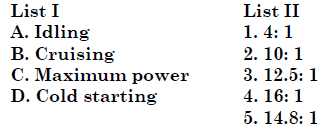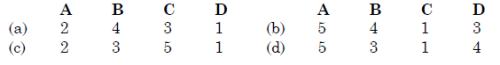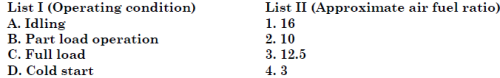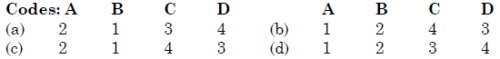# SI And CI Engines - MCQ Test 1

## 20 Questions MCQ Test RRB JE for Mechanical Engineering | SI And CI Engines - MCQ Test 1

Description
This mock test of SI And CI Engines - MCQ Test 1 for Mechanical Engineering helps you for every Mechanical Engineering entrance exam. This contains 20 Multiple Choice Questions for Mechanical Engineering SI And CI Engines - MCQ Test 1 (mcq) to study with solutions a complete question bank. The solved questions answers in this SI And CI Engines - MCQ Test 1 quiz give you a good mix of easy questions and tough questions. Mechanical Engineering students definitely take this SI And CI Engines - MCQ Test 1 exercise for a better result in the exam. You can find other SI And CI Engines - MCQ Test 1 extra questions, long questions & short questions for Mechanical Engineering on EduRev as well by searching above.
QUESTION: 1

Solution:

Ans. (b)

QUESTION: 2

### For a conventional S.I. engine, what is the value of fuel-air ratio in the normal operating range?

Solution:

Ans. (a) (A/F) for maximum power = 12 and (A/F) for Stoichiometric = 14.5
(A/F) for maximum fuel economy = 16.

QUESTION: 3

### The air fuel ratio for idling speed of an automobile patrol engine is closer to

Solution:
QUESTION: 4

Match List I (S.I. Engine Operational Mode) with List II (Air fuel Ratio by Mass) and select the correct answer:Solution:

Ans. (a)

QUESTION: 5

Match List I with List II and select the correct answer.Solution:

Ans. (a)

QUESTION: 6

Stoichiometric air-fuel ratio by volume for combustion of methane in air is:

Solution:

Ans. (c)

QUESTION: 7

Velocity of flame propagation in the SI engine is maximum for a fuel-airmixture which is

Solution:

Ans. (a) When the mixture is nearly 10% richer than stoichiometric (fuel-air ratio = 0.08 i.e. air-fuel ratio 12.5:1) the velocity of flame propagation is maximum and ignition lag of the end gas is minimum.

QUESTION: 8

Which of the following cannot be caused by a hot spark plug?
1. Pre-ignition
2. Post-ignition
3. Detonation
4. Run-on-ignition
Select the correct answer using the code given below:

Solution:

Ans. (b)

QUESTION: 9

Match List I with List IT and select the correct answer using the codes given below the lists:
List I                                                               List II
(S.l. Engine problem)                       (Characteristic of fuel responsible for the problem)
A. Cold starting                                1. Front end volatility
B. Carburetor icing                           2. Mid-range volatility
C. Crankcase dilution                      3. Tail end volatility
Code: A B C                         A B C
(a) 1 2 3                    (b) 1 3 2
(c) 2 3 1                    (d) 3 1 2

Solution:

Ans. (a)

QUESTION: 10

Which of the following factors increase detonation in the SI engine?
2. Increased speed.
3. Increased air-fuel ratio beyond stoichiometric strength
4. Increased compression ratio.
Select the correct answer using the codes given below:

Solution:

Ans. (d) Detonation in the S.I. engines is increased by increasing spark advance and
increased compression. The increased speed and lean mixtures do not have much influence.

QUESTION: 11

The tendency of petrol to detonate in terms of octane number is determinedby comparison of fuel with which of the following?

Solution:

Ans. (b)

QUESTION: 12

For minimizing knocking tendency is SI engine, where should the space plug be located?

Solution:

One of the fixes to engine knocking is to change the positioning of the spark plug. Since engine is a result of pre-mature burning of fuel-air mixture, the ignition mechanism must have correct spacing where the volume would be maximized. In this case, the spark plug must be placed near the exhaust valve to have greater travel distance of spark to the air-fuel mixture. This would produce less pressure to the chamber, and since the volume of the chamber is maximized, even burning of fuel-air mixture can be observed.

QUESTION: 13

In spark ignition engines knocking can be reduced by:

Solution:

Ans. (c)

QUESTION: 14

Which of the following are the assumptions involved in the auto-ignition theory put forth for the onset of knock in SI engines?
1. Flame velocity is normal before the onset of auto ignition.
2. A number of end-gas elements auto ignite simultaneously.
3. Preflame reactions are responsible for preparing the end-gas to ignite.
Select the correct answer using the codes given below:

Solution:

Ans. (d)

QUESTION: 15

Assertion (A): Self-ignition temperature of the end charge must be higher to prevent knocking of an SI engine.
Reason (R): Higher compression ration increases the temperature of the air-fuelmixture.

Solution:

Ans. (d) Self ignition temperature of end of charge must be lower to prevent knocking.
Higher compression ratio increases temperature of air fuel mixture. Thus A is false and R is correct.

QUESTION: 16

Match List I with List II, in respect of SI engines, and select the correct answer by using the codes given below the lists:
List I                                                            List II
A. Highest useful compression ratio         1. Ignitable mixture
B. Dopes                                                   2. Knock rating of fuels
C. Limiting mixture strength                      3. Detonation
D. Delay period                                         4. Chain of chemical reactions in combustion chamber
Codes: A B C D                        A B C D
(a) 2 3 1 4                    (b) 3 2 1 4
(c) 2 3 4 1                    (d) 3 4 2 1

Solution:

Ans. (b)

QUESTION: 17

Consider the following statements
1. Octane rating of gasoline is based on iso-octane and iso-heptane fuelswhich are paraffin
3. Ethylene di-bromide is added as scavenging agent to remove lead depositson spark plugs.
4. Surface ignition need not necessarily cause knocking.
Which of these statements are correct?

Solution:

Ans. (b) Octane rating of gasoline is based on iso-octane and n-heptane fuels which are paraffin so 1 is wrong.

QUESTION: 18

The volumetric efficiency of a well designed SI engine is in the range of

Solution:

Ans. (d)

QUESTION: 19

Knocking in the SI engine decreases in which one of the following orders of combustion chamber designs?

Solution:

Ans. (c)

QUESTION: 20

Which of the following combustion chamber design features reduce(s) knocking in S.I. engines?
1. Spark plug located near the inlet valve.